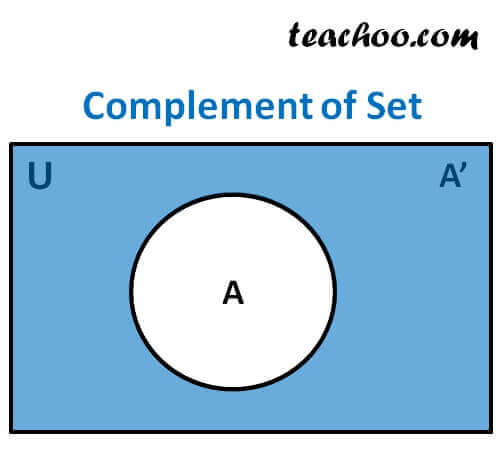Complement of set

Chapter 1 Class 11 Sets
Concept wise

Complement of set A means

all elements of Universal set which are not in A

It is denoted by A’

Let A = {1, 2, 3, 4} , B = {3, 4, 5, 6}, C = {6, 7, 8}

and Universal set = U = {1, 2, 3, 4, 5, 6, 7, 8, 9, 10}

A’ = U – A

A’ = { 1, 2, 3, 4 , 5, 6, 7, 8, 9, 10} – { 1, 2, 3, 4 }

A’ = {5, 6, 7, 8, 9, 10}## Properties of Complement

1. Complement laws:
(i) A ∪ A′= U (ii) A ∩ A′= ∅
2. De Morgan’s law :
(i) (A ∪ B)´ = A′ ∩ B′ (ii) (A ∩ B)′= A′ ∪ B′
3. Law of double complementation :
(A′)′= A
4. Laws of empty set and universal set
∅′ = U and U′= ∅

Learn in your speed, with individual attention - Teachoo Maths 1-on-1 Class